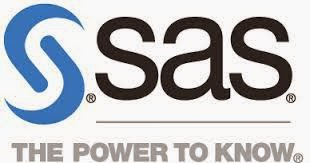## Posts

Showing posts with the label Excel analytics

### Excel: 10 Key Topics You Need to LearnThe below-listed topics help you get a solid footing in Excel Analytics. Just practice these 10 topics step by step and by completing all, you will be an expert in Excel. 10 Top Excel Topics Tables in Excel  Grabbing data from external sources  Cleaning data with functions  Working with Pivot tables  Writing Formulae for Pivot tables  Pivot Charts  How to use database functions  How to use statistics  Inferential Statistics  Descriptive statistics Also Read : 5 Tips why macros need in Excel

### Excel Analytics: The complete best explained Free Tutorials

Can you do analytics—either kind—using Excel? Sure. Excel has a large array of tools that bear directly on analytics, including various mathematical and statistical functions that calculate logarithms, regression statistics, matrix multiplication and inversion, and many of the other tools needed for different kinds of analytics. But not all the tools are native to Excel.  For example, some situations call for you to use logistic regression: a technique that can work much better than ordinary least-squares regression when you have an outcome variable that takes on a very limited range of values, perhaps only two. Odds ratios are the workhorses of logistic regression, but although Excel offers a generous supply of least-squares functions, it doesn’t offer a maximum likelihood odds ratio function.  The below list makes you strong in Excel analytics Microsoft Excel 2010 Data Analysis With Functions Analytics with Excel - Book Cloud data analytics from Excel Quantitative Analysis

### A Powerful Video to Import MS Excel into SAS (Part-1)#A Powerful Video to Import MS Excel into SAS: SAS is a Statistical Analytical Software. The below video shows how MS-EXCEL data import into SAS for analysis.

### Course Topics You Need to Know Before You Take Course on Excel

Hey, you want to be master in Excel. There are 4 parts in this course. These contents cover all the functionalities you need to work with Excel. Excel is one of the tools to be used in data analytics Why I have given contents means these you must ask your tutor if present in the course or not. This list useful to start a career in analytics. List of Excel Course Topics Part-1 - Importing Data from other sources Import or Export data from multiple data sources Part-2 - Converting data Excel ready Formatting the data understand by EXCEL. Part-3 - Data Mining Formulas you need for Data cleaning. Part-4- Excel Data Analysis Tools Data analysis using statistical methods, Charts and Pivot Tables